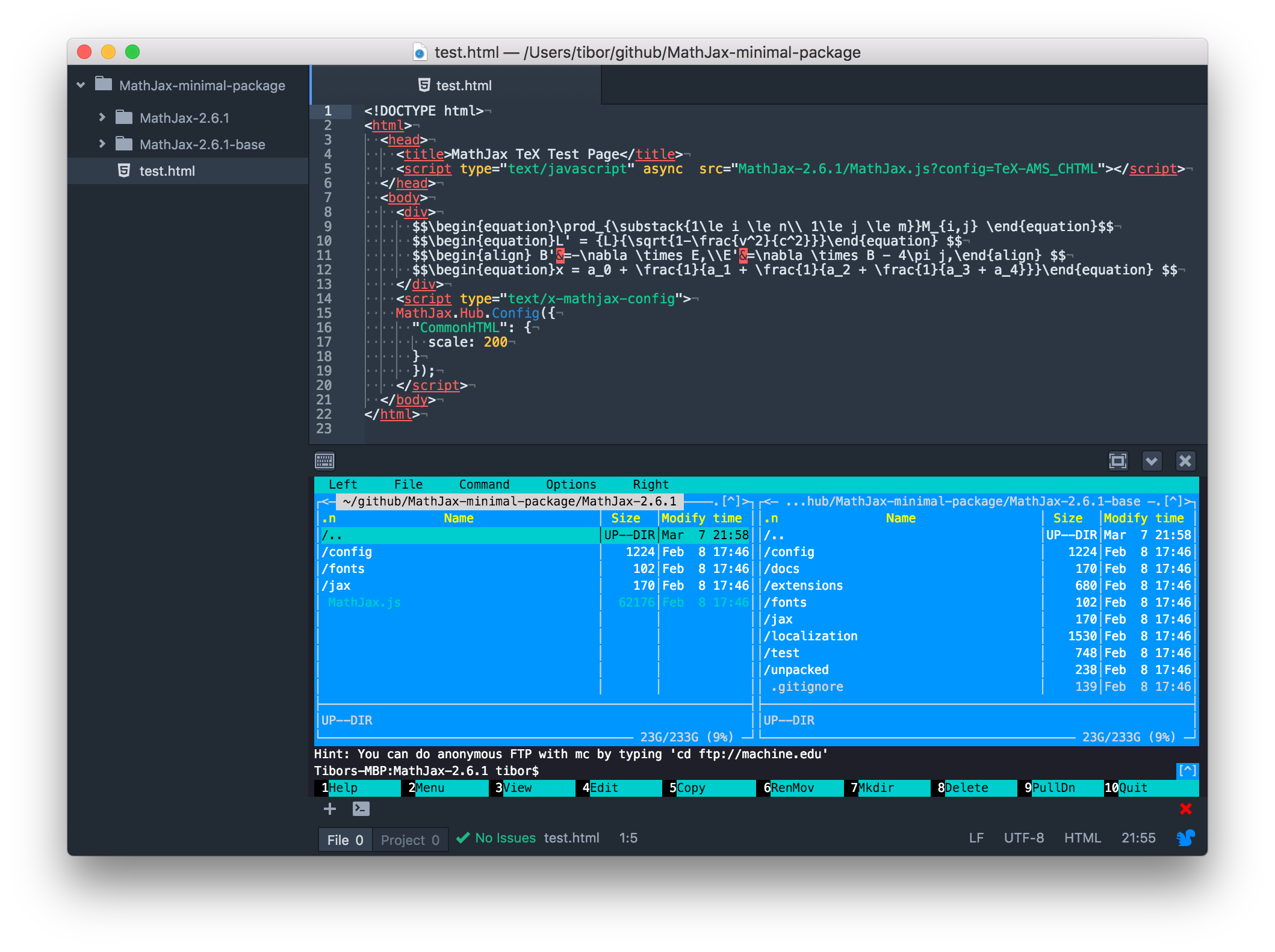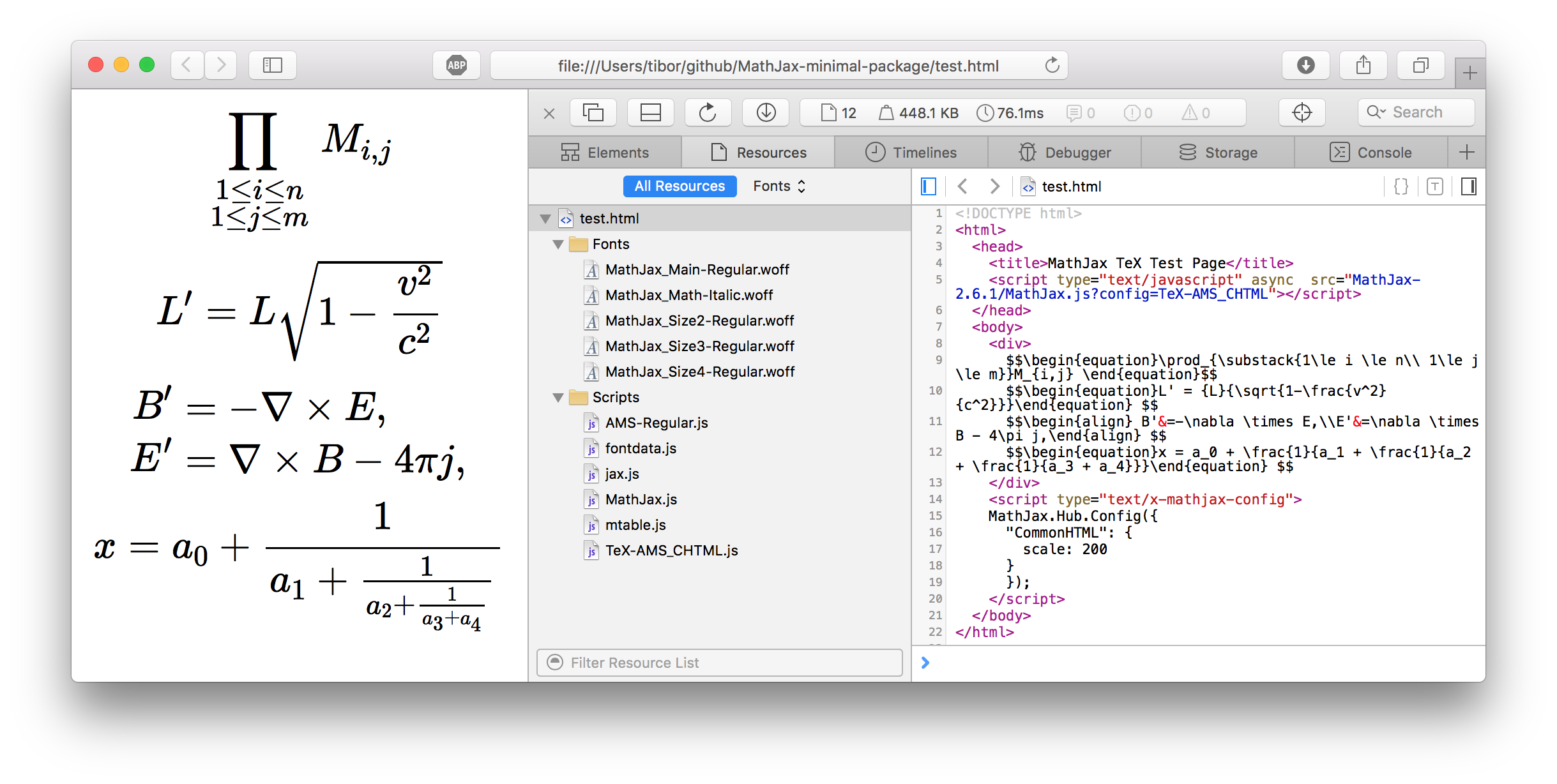Minimal MathJax package
2016. 03. 07.
MathJax is a pretty big javascript package, but you can make it work after you stripped off most of the files. The final package size could be around 623KB.

In the [previous article](/articles/programming/ios-mathjax-integration) we have created a working MathJax based math rendering iOS app. In this article I will show you how you can shrink down the original 180MB MathJax package into an about 623KB package. This is 99.6% size reduction.

Component Version
MathJax 2.6.1

MathJax supports a very wide range of input and output methods. That is the reason that it is big package. Therefore the most important thing in MathJax size reduction is to decide what input and output method you want to support. In this way you only need to have those sources that implement that methods by throwing away the rest of the package.

In my example, I wanted to have LaTEX input and Common HTML output.

# The reducing method ¶

The best way to reduce your MathJax package is to create a simple template page, that uses MathJax as you would use it anyway, then remove almost every package content, and then add the ones that it tries to load but fails because the file isn’t present. In this way you will end up a package that contains just the right resources to render your equations.

I started with a simple html page containing a basic MathJax setup:

<!DOCTYPE html>
<html>
<title>MathJax TeX Test Page</title>
<script type="text/javascript" async  src="MathJax-2.6.1/MathJax.js?config=TeX-AMS_CHTML"></script>
<body>
<div>
$$\begin{equation}\prod_{\substack{1\le i \le n\\ 1\le j \le m}}M_{i,j} \end{equation}$$
$$\begin{equation}L' = {L}{\sqrt{1-\frac{v^2}{c^2}}}\end{equation}$$
\begin{align} B'&=-\nabla \times E,\\E'&=\nabla \times B - 4\pi j,\end{align}
$$\begin{equation}x = a_0 + \frac{1}{a_1 + \frac{1}{a_2 + \frac{1}{a_3 + a_4}}}\end{equation}$$
</div>
<script type="text/x-mathjax-config">
MathJax.Hub.Config({
"CommonHTML": {
scale: 200
}
});
</script>
</body>
</html>


The easiest way I find to put back the necessary resources to the package is to copy the full package into a separated directory, then in a two pane file manager, put back the requested files. You can use your browser’s inspector view to find out what is missing.Fig 1: Editing the html file while putting back the necessary resources.Fig 2: Discovering missing resources in the browser's inspector.

This html test page renders the following equations:Fig 3: Testing the MathJax package with a small html file.

If my reduced MathJax package can render this equations, it shouldn’t be in trouble render all of my possible further equations. The html page above runs fine with teh following resource tree lising. This reduced package takes up 623KB on disk.

MathJax-2.6.1
├── MathJax.js
├── config
│   └── TeX-AMS_CHTML.js
├── fonts
│   └── HTML-CSS
│       └── TeX
│           └── woff
│               ├── MathJax_AMS-Regular.woff
│               ├── MathJax_Math-Italic.woff
│               ├── MathJax_Size2-Regular.woff
│               ├── MathJax_Size3-Regular.woff
│               └── MathJax_Size4-Regular.woff
└── jax
└── output
└── CommonHTML